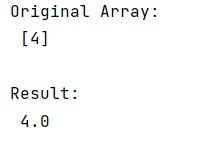# Convert list or NumPy array of single element to float

Learn, how to Convert list or NumPy array of single element to float in Python?
Submitted by Pranit Sharma, on February 26, 2023

NumPy is an abbreviated form of Numerical Python. It is used for different types of scientific operations in python. Numpy is a vast library in python which is used for almost every kind of scientific or mathematical operation. It is itself an array which is a collection of various methods and functions for processing the arrays.

## Converting a list or array of single element to float

Suppose that we are given a list or a numpy array with a single element and a function that can accept either a list or a numpy array and we need to return a float value from this function.

For this purpose, we will Just access the first item of the list/array, using the index access and the index 0, this will be an int since that was what we inserted in the first place. If we want to convert it into a float, we can call the defined function where we can write a code to apply float() on the item.

Let us understand with the help of an example,

## Python code to convert list or NumPy array of single element to float

```# Import numpy
import numpy as np

# Creating a numpy array
arr = np.array()

# Display original array
print("Original Array:\n",arr,"\n")

# Defining a function
def fun(item):
return float(item)

# Calling the function with the
# first element of the array
res = fun(arr)

# Display result
print("Result:\n",res)
```

Output:Languages: » C » C++ » C++ STL » Java » Data Structure » C#.Net » Android » Kotlin » SQL
Web Technologies: » PHP » Python » JavaScript » CSS » Ajax » Node.js » Web programming/HTML
Solved programs: » C » C++ » DS » Java » C#
Aptitude que. & ans.: » C » C++ » Java » DBMS
Interview que. & ans.: » C » Embedded C » Java » SEO » HR
CS Subjects: » CS Basics » O.S. » Networks » DBMS » Embedded Systems » Cloud Computing
» Machine learning » CS Organizations » Linux » DOS
More: » Articles » Puzzles » News/Updates